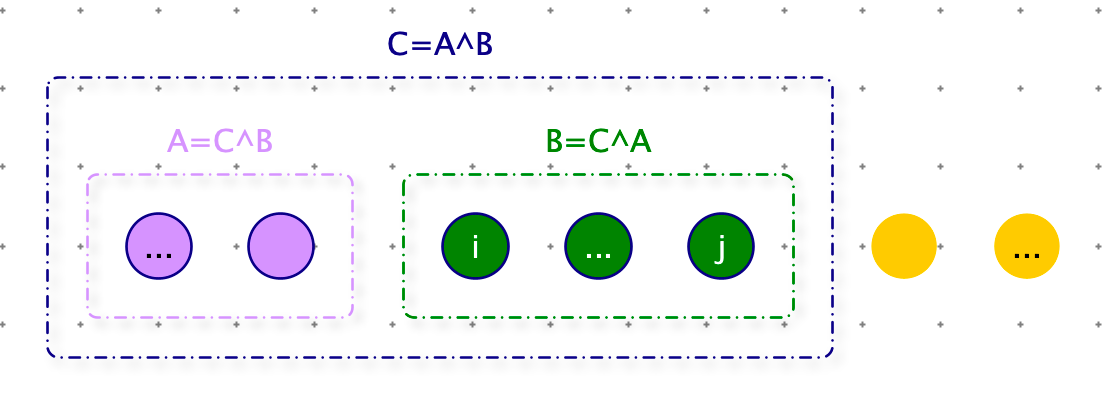V1

2022/11/03阅读：16主题：红绯

# 子数组最大异或和

【举例】

arr = 【3】

arr = 【3，-28，-29，2】

``import sysdef max_xor(arr):    if not arr: return 0    res = -sys.maxsize    for i in range(len(arr)):        for j in range(i, len(arr)):             # 窗口:arr[i,j+1],计算窗口内数据的异或和            xor = 0            for k in range(i, j + 1):                xor ^= arr[k]            res = max(res, xor)    return res``

1. 归零率：A ^ A = 0
2. 恒等率：A ^ 0 = A

#``def max_xor1(arr):    if not arr: return 0   # 前缀异或和    prefix_sum = [arr]    for i in range(1, len(arr)):        prefix_sum.append(arr[i] ^ prefix_sum[-1])    res = -sys.maxsize    for i in range(len(arr)):        s = 0 if i == 0 else prefix_sum[i - 1]        for j in range(i, len(arr)):            # 窗口:arr[i,j+1]            xor = prefix_sum[j] ^ s            res = max(res, xor)    return res``

• arr[0..5] 与 0 结合表示：arr[0...5] 子数组的异或和
• arr[0..5] 与 arr 结合表示：arr[1...5] 子数组的异或和
• arr[0..5] 与 arr[0...1] 结合表示：arr[2...5] 子数组的异或和
• arr[0..5] 与 arr[0...2] 结合表示：arr[3...5] 子数组的异或和
• ...

``# 将所有的前缀异或和，加入到 NumTrie，并按照前缀树组织class NumTrie:    def __init__(self):        self.root = Node()    def add(self, num):        cur = self.root        # move 向右位移多少位        for move in range(31, -1, -1):            # 获取对应位上的数字（0 或者 1）            path = (num >> move) & 1            cur.nexts[path] = cur.nexts[path] if cur.nexts[path] else Node()            cur = cur.nexts[path]    # num 最希望遇到的路径，结果返回：最大的异或和    # 时间复杂度：O(32)    def max_xor(self, num):        cur = self.root        # 返回值(num ^ 最优选择)        res = 0        for move in range(31, -1, -1):            # 获取对应位上的数字（0 或者 1）            path = (num >> move) & 1            # sum 该位的状态，最期待的路径（如果sum 位是0，期待path =1，否则 path = 0）            # 注意：如果是符号位是 1（负数），期待 path = 1，异或后是 0（正数）            #      如果是符号位是 0（正数），期待 path = 0，异或后是 0（正数）            best = path if move == 31 else path ^ 1            # 最期待走的路径  --> 实际路径            best = best if cur.nexts[best] else best ^ 1            # 注意：本代码是 python，左移 31 位不会变为负数，python 会将 int 转为 long 变为更大的数            # 如果是 java：res |= (path ^ best) << move            tmp = 1            if move == 31 and num < 0:                tmp = -1            res |= tmp * (path ^ best) << move            cur = cur.nexts[best]        return res``

``def max_xor2(arr):    if not arr: return 0    res = -sys.maxsize    trie = NumTrie()    trie.add(0)    # 一个数没有时，异或和为 0    xor = 0    for i in range(len(arr)):        # xor 等于 0 ~ i 异或和        xor ^= arr[i]        # trie 装着所有：一个数也没有（0），0~1，0~2，0~3...0~i-1 的异或和        res = max(res, trie.max_xor(xor))        trie.add(xor)    return res``

``import randomdef check():    max_value = 10    for i in range(100):        arr = [int(random.random() * max_value) - int(random.random() * max_value) for _ in               range(int(random.random() * max_value))]        res = max_xor(arr)        res1 = max_xor1(arr)        res2 = max_xor2(arr)        if res != res1 or res != res2:            print(i, "ERROR", arr, "res=", res, "res1=", res1, "res2=", res2)    print("NICE")``V1